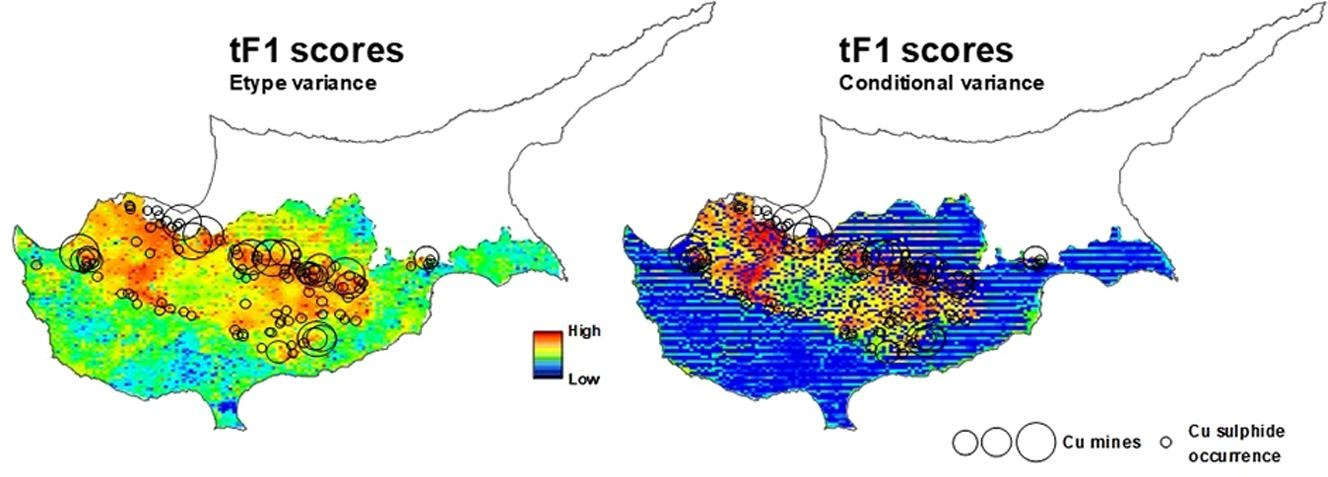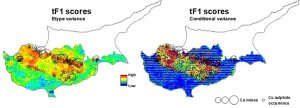# Simulated-multifractal models: A futuristic review of multifractal modeling in geochemical anomaly classification

Dr. Behnam Sadeghi, from EarthByte Group (USYD), has published a new review paper in “Ore Geology Reviews”. Sadeghi et al. (2015) proposed simulated-fractal models for the first time, which is based on the combination of geostatistical simulation models and mathematical fractal/multifractal modeling to classify geochemical anomalies. Based on that research, several other models have been developed. In this review, Behnam reviews the traditional fractal/multifractal classification models, and provides new simulated multifractal models. All such models are based on both frequency and Bayesian frameworks, therefore the final models (in 2D and 3D) would be strongly helpful with robust decision-makings under uncertainty.

Abstract

Various statistical methods, including fractal/multifractal techniques, have been applied to clustering of geochemical samples based on their elemental concentrations. Most classification models provide a single classification map whose precision (stability) and accuracy are unknown. Classification models based on more than one approach permit assessment of such uncertainty, but the effect of different assumptions in the model may be difficult to assess. Alternatively, the precision can be assessed using simulation methods on a given modelling approach, such as sequential Gaussian simulation (SGSIM) with a large number of realizations with changes in classification (number of clusters or allocation of samples to specific clusters). In this research, novel robust simulated-multifractal classification models have been introduced and reviewed based on several traditional as well as newly established multifractal models for further consideration in geochemical anomaly classification.

Full link to this paper is: https://www.sciencedirect.com/science/article/pii/S0169136821005370?via%3Dihub